Auto Populate Cells Based on Dropdown Box Selection

This post will guide you how to create a drop down list and how to auto fill cells in Excel based on a dependent drop down list. When selecting a value from the drop down list, then it should be able to populate the value in another corresponding cell.

For examples, you have created a drop down list based on the values in range B2:B4, and when you select any value from the drop down list, the corresponding value in range c2:C4 should be populated in another cell automatically.

Auto Populate Cells Based on Dropdown Box Selection

#1 select one cell that will be holding the drop down list. Such as: B1

#2 Go to DATA tab, click Data Validation command under Data Tools group.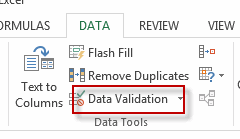#3 the Data Validation window will appear.

#4 change the Allow: value to “List” from the drop-down list under Validation criteria section. Then select range B2:B4 as Source data.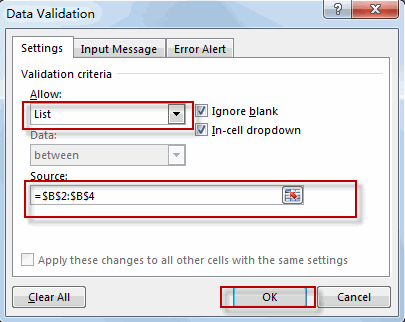#5 the drop down list has been created.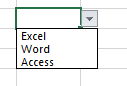#6 type the below formula in another cell D6, press Enter key.

=VLOOKUP(B6,B2:C4,2,FALSE)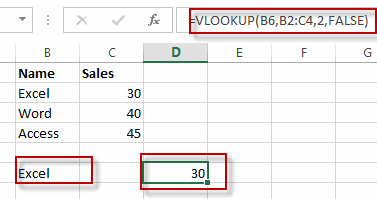#7 select another value “Word” from the drop-down list in Cell B6, you will see that the corresponding value in Cell D6 will be populated automatically.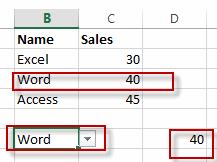Related Posts

Filter Data by Column and Sort by Row

This post will guide you how to use the FILTER function to filter a value by column and then sort data by row in Microsoft Excel. You can use the following formula based on the SORT function in combination with ...

Filter And Transpose Data From Horizontal To Vertical

This post will show you how to use Filter function and in combination with Transpose function to filter data from horizontal and transpose data as vertical in Microsoft Excel. You can refer to the below general formula based on TRANSPOSE ...

VLOOKUP Formula | Faster Trick with 2 VLOOKUPS

This post will guide you how to use 2 VLOOKUPS function to looking up data entries from a given range of cells in Microsoft Excel. VLOOKUP with 2 lookups can be faster than a single VLOOKUP in certain scenarios. The ...

Extract Unique Items From A List

This post will guide you how to extract unique itmes from a given list in Microsoft Excel. How to create a newly formula to get unique values from a range cells in Excel. The unique list of items is the ...

Extract substring In Excel

This post will guide you how to use Excel's MID function is a quick and easy way to extract pieces from your text. Use the Excel formula to extract a substring with MID. Note: If you want to extract just ...

How to Use 3D SUM Multiple Worksheets

To sum a range of numbers is straightforward for most Excel users, but do you know how to establish a 3D reference to total the same range of numerous sheets. In this post, I will present the steps for this ...

Extract all the matches with helper Column

With Excel's powerful functions IF, INDEX, and MATCH, we can find exactly what you're looking for with a few clicks of the mouse. This step-by-step tutorial will show how easy it is to extract data using these tools and more! ...

If Cell is This Value or That Value

IF function is frequently used in Excel worksheet to return you expect “true value” or “false value” based on the result of logical test. If you want to see if a cell is A or B, and if one of ...

If Value is Greater Than A Certain Value

IF function is frequently used in Excel worksheet to return you expect “true value” or “false value” based on the logical test result. If you want to see if a value in one cell is greater than a specific value, ...

If Cell is Not Blank

IF function is frequently used in Excel worksheet to return you expect “true value” or “false value” based on the result of created logical test. If you want to see if a cell is blank or not, and leave some ...

Sidebar